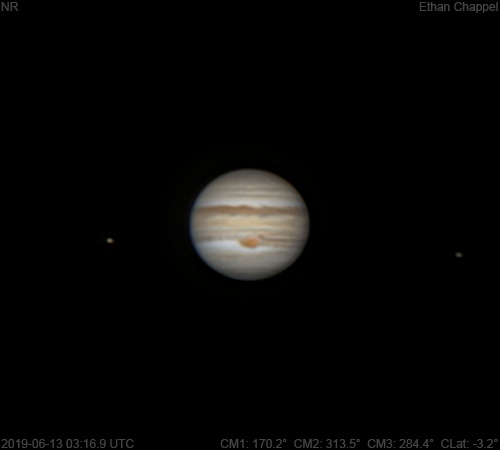# Jupiter 2019-06-13 03:16 UTC

CM1: 170.20°

CM2: 313.50°

CM3: 284.40°

CLat: -3.20°

Description

Another night starting with a test of the sky conditions with the 90mm Mak before bringing out a bigger scope. Conditions were not as good as the previous session.

Notes:

• White Oval Z is just past the central meridian.
• The Equatorial Zone is orange.
• The Great Red Spot is on the central meridian.
• Clouds from the South Equatorial Belt are circulating around the GRS and into the South Tropical Zone to the east.
• Io and Europa are left and right of the planet respectively.

Equipment

ZWO ASI224MC

Orion Apex 90mm

Logs
```FireCapture v2.6  Settings
------------------------------------
Observer=Ethan Chappel
Camera=ZWO ASI224MC
Filter=RGB
Profile=Jupiter
Diameter=45.96"
Magnitude=-2.62
CMI=171.0° CMII=314.2° CMIII=285.2°  (during mid of capture)
FocalLength=1150mm (F/4)
Resolution=0.67"
Filename=2019-06-13-0318_3-EC-RGB-Jup.ser
Date=2019_06_13
Start=03_17_05.625
Mid=03_18_20.627
End=03_19_35.629
Start(UT)=03_17_05.625
Mid(UT)=03_18_20.627
End(UT)=03_19_35.629
Duration=150.004s
Date_format=yyyy_MM_dd
Time_format=HH_mm_ss
LT=UT -6h
Frames captured=9594
File type=SER
Binning=no
Bit depth=8bit
Debayer=yes
DebayerAlgorithm=NearestNeighbor
BayerPattern=RG
ROI=364x248
ROI(Offset)=0x0
FPS (avg.)=63
Shutter=7.000ms
Gain=280 (46%)
SoftwareGain=10 (off)
AutoHisto=75 (off)
AutoExposure=off
AutoGain=off
HighSpeed=off
WBlue=95 (off)
Gamma=50 (off)
FPS=100 (off)
Brightness=1 (off)
USBTraffic=100 (off)
HardwareBin=off
WRed=52 (off)
Histogramm(min)=0
Histogramm(max)=128
Histogramm=50%
Noise(avg.deviation)=0.09
AutoAlign=false
PreFilter=none
Limit=150 Seconds
Sensor temperature=32.5°C
FireCapture v2.6  Settings
------------------------------------
Observer=Ethan Chappel
Camera=ZWO ASI224MC
Filter=RGB
Profile=Jupiter
Diameter=45.96"
Magnitude=-2.62
CMI=169.3° CMII=312.5° CMIII=283.5°  (during mid of capture)
FocalLength=1150mm (F/4)
Resolution=0.68"
Filename=2019-06-13-0315_5-EC-RGB-Jup.ser
Date=2019_06_13
Start=03_14_18.897
Mid=03_15_33.901
End=03_16_48.905
Start(UT)=03_14_18.897
Mid(UT)=03_15_33.901
End(UT)=03_16_48.905
Duration=150.008s
Date_format=yyyy_MM_dd
Time_format=HH_mm_ss
LT=UT -6h
Frames captured=9594
File type=SER
Binning=no
Bit depth=8bit
Debayer=yes
DebayerAlgorithm=NearestNeighbor
BayerPattern=RG
ROI=364x248
ROI(Offset)=0x0
FPS (avg.)=63
Shutter=7.000ms
Gain=280 (46%)
SoftwareGain=10 (off)
AutoHisto=75 (off)
AutoExposure=off
AutoGain=off
HighSpeed=off
WBlue=95 (off)
Gamma=50 (off)
FPS=100 (off)
Brightness=1 (off)
USBTraffic=100 (off)
HardwareBin=off
WRed=52 (off)
Histogramm(min)=0
Histogramm(max)=132
Histogramm=51%
Noise(avg.deviation)=0.09
AutoAlign=false
PreFilter=none
Limit=150 Seconds
Sensor temperature=32.3°C
```